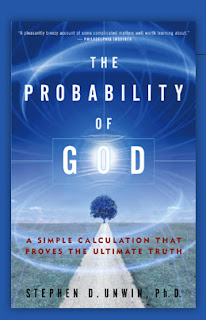## Thursday, January 17, 2008

### The probability of GodThe Notices of the American Mathematical Society features a twin book review this month: Stephen Unwin's notorious 2004 work, The Probability of God: A simple calculation that proves the ultimate truth, and Steven Brams's more recent work, Superior Beings: If they exist, how would we know?

Unwin's book uses Bayes's theorem to calculate a 67% probability for the existence of God! One popular form of Bayes's theorem is the following:

P(H|E) = P(E|H)P(H)/(P(E|H)P(H) + P(E|~H)P(~H))

H denotes a hypothesis, ~H denotes the negation of the hypothesis, E denotes a piece of evidence, P(H) denotes the prior probability of the hypothesis, P(~H) denotes the prior probability of the falsity of the hypothesis, P(E|H) denotes the conditional probability of the piece of evidence E given the truth of the hypothesis H, P(E|~H) denotes the conditional probability of the piece of evidence given the falsity of the hypothesis, and P(H|E) denotes the conditional probability of the hypothesis given the piece of evidence E.

The numerator on the right-hand side here gives the probability that the piece of evidence could be explained by the hypothesis H, while the denominator gives the probability that the piece of evidence could be explained by anything, (in fact, the denominator is the same as P(E), the unconditional probability of E). The ratio of these two values gives the posterior probability of H, given the piece of evidence E.

The basic idea is that the prior probability of the hypothesis P(H) is updated to P(H|E) in light of new evidence E. The process is iterated for each new piece of evidence. In the case of interest here, H is the hypothesis that God exists. Unwin begins by assigning a 50% prior probability P(H) that God exists, and a 50% prior probability P(~H) that the hypothesis is false. It seems, then, that any questions over whether the hypothesis is even meaningful, are not to be considered.

Unwin then considers six pieces of evidence, and plucks, out of thin air, conditional probabilities P(E|H)/P(E) for such evidence given the existence of God, relative to the unconditional probability P(E) of each piece of evidence. Unwin specifies that for E = the existence of goodness, P(E|H)/P(E) = 10; for E = existence of moral evil, P(E|H)/P(E) = 0.5; for E = existence of natural evil, P(E|H)/P(E) = 0.1; for E = intranatural miracles (prayers), P(E|H)/P(E) = 2; for E = extranatural miracles (resurrection), P(E|H)/P(E)= 1; and for E = religious experiences P(E|H)/P(E) = 2. Given that the denominator from the expression of Bayes's theorem above is the same as P(E), Bayes's theorem simplifies to:

P(H|E) = P(H) × P(E|H)/P(E)

By iterating this calculation six times, for each piece of evidence, Unwin updates the probability for the existence of God to 67%. Michael Shermer assigns alternative values to the conditional probabilities, and uses Bayes's theorem to calculate that the probability of God is 2%.

As Shermer comments, "all such scientistic theologies are compelling only to those who already believe. Religious faith depends on a host of social, psychological and emotional factors that have little or nothing to do with probabilities, evidence and logic. This is faith's inescapable weakness. It is also, undeniably, its greatest power."

Selena Dreamy said...

It seems, then, that any questions over whether the hypothesis is even meaningful, are not to be considered.

Precisely!

God is a conjecture,” said Nietzsche, “but I should like your conjecturing restricted to the conceivable.” Time was, when medieval theologians debated the question of how many angels could dance on the tip of a pin, and this particular application of Bayes’ theorem rather reminds me of the proverb about people in glass houses.

One is as bad as the other!

To me it seems extraordinary, that after some 500 years of enlightenment and the advent of quantum physics, humans still consider the existence of divine beings or esoteric phenomena as something independent of the human mind. Frankly, by a reverse kind of paradox, you might as well calculate the probability of ghosts.

God is a metaphor. And not some absurd oriental fantasy, let an old hippie with bad teeth and so much beard protruding from his jowls that he looks as if he is peering out of a yak's ass.

Dreamy

Gordon McCabe said...

Nice heels, Selena!

Selena Dreamy said...

The shortcoming in this case, Gordon, of Bayes’ Theorem, is that one cannot reliably calculate the odds of a particular entity, unless one either understands the nature of the thing - that is, can properly distinguish and quantify all the variables involved - or has sufficient experimental data from which to draw phenomenological conclusions.

But when the question involves the “heels” of my stilettos, we need with confidence do neither, since all we require is an elementary visual understanding of the variables involved ....

...and none whatsoever regarding statistics!

DreamyAnonymous said...

What's God a metaphor for, Selena?